ConceptDraw
DIAGRAM 14

# Bar Diagram Math

ConceptDraw DIAGRAM extended with Divided Bar Diagrams solution from Graphs and Charts area of ConceptDraw Solution Park is the best software for quick and simple drawing the Divided Bar Diagrams and Bar Diagram Math.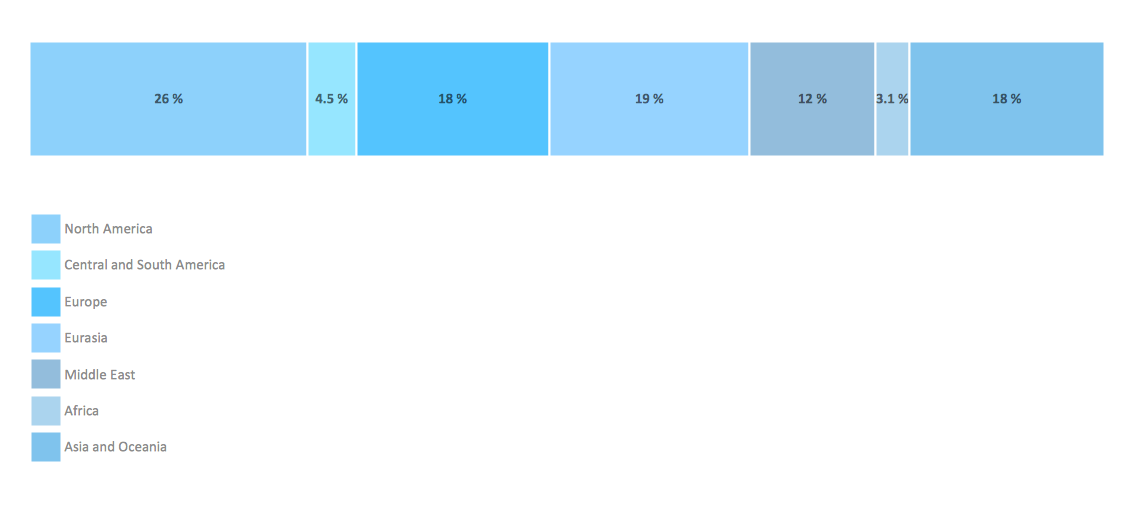Example 1. Bar Diagram Math - Global natural gas consumption

Divided Bar Diagrams solution includes wide variety of Bar Diagram Math examples, templates and samples which are available from ConceptDraw STORE. They can help you in your work - you can use them as the base for your own Bar Diagram Math. All that you need - simply change the details of desired sample, or fill template for your needs.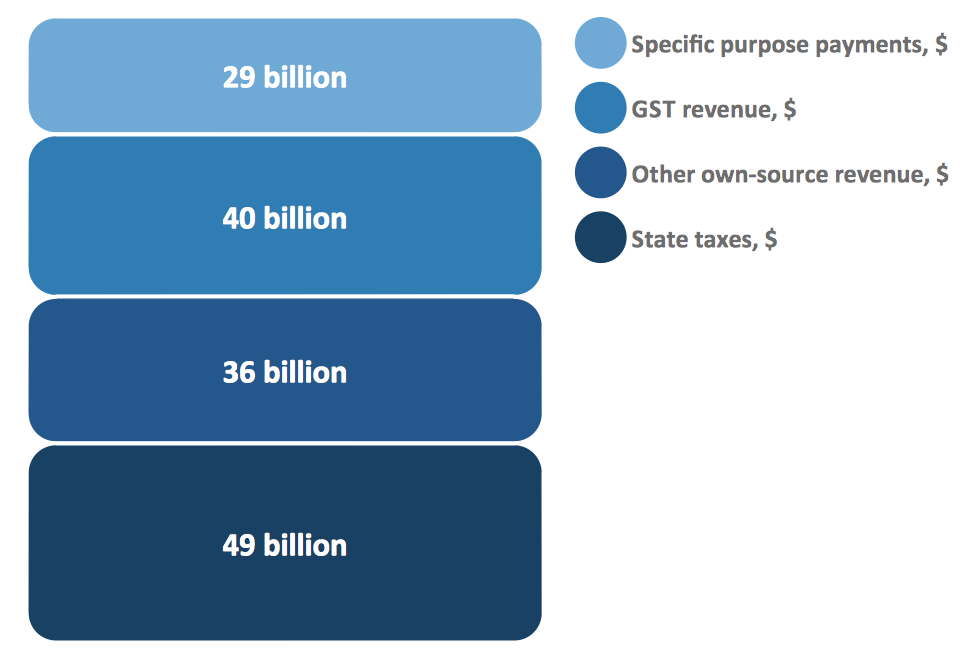Example 2. Bar Diagram Math - Composition of state government revenue

Divided Bar Diagrams solution contains also Divided Bar Diagrams library with wide variety of predesigned vector objects that will be useful for creating the Bar Diagram Math in minutes.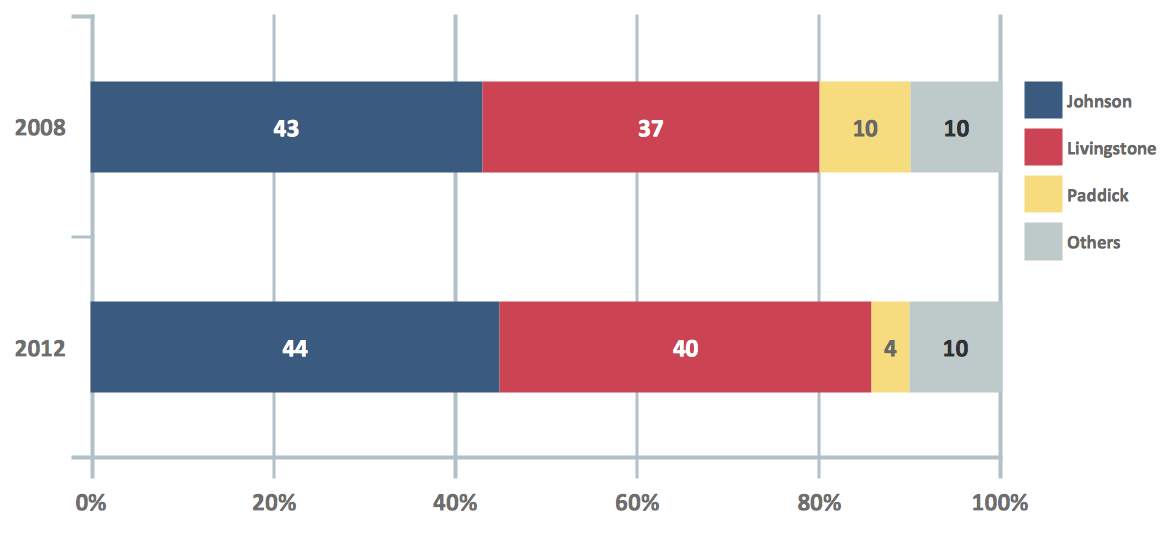Example 3. Bar Diagram Math - London election results

The examples you see on this page were created in ConceptDraw DIAGRAM using the objects from the Divided Bar Diagrams library of Divided Bar Diagrams solution. These samples demonstrate the solution's capabilities and the professional results you can achieve. An experienced user spent 10 minutes creating every Bar Diagram Math of represented on this page.

The Bar Diagram Math and Divided Bar Diagrams produced with ConceptDraw DIAGRAM is vector graphic documents and is available for reviewing, modifying, converting to a variety of formats (image, HTML, PDF file, MS PowerPoint Presentation, Adobe Flash or MS Visio), printing and send via e-mail in one moment.

### Components of ER Diagram →

It is easy to recreate any informational system structure using diagrams. There are three main components of any ER diagram: entity, attribute and relationship. Basing on these three components, one can define other, less used elements, such as weak entity or relationship, derived attribute, recursive relationship etc. This is the set of graphic elements of ERD Chen's notation. This ERD notation is used to represent an entity–relationship models. It involves the set of geometric forms: rectangles - depicting entities, ovals - representing attributes and diamonds depicting relationships assigned for first-class objects, that can have relationships and attributes of their own. Connections are displayed with arrowed lines. It is known that the Chen's ERD notation is used to show a detailed view of entities and relationships. ConceptDraw Entity-Relationship Diagram solution from the Software Development section of Solution Park provides the ability to create ERD of database structure for software development purposes using the Chen’s notation elements.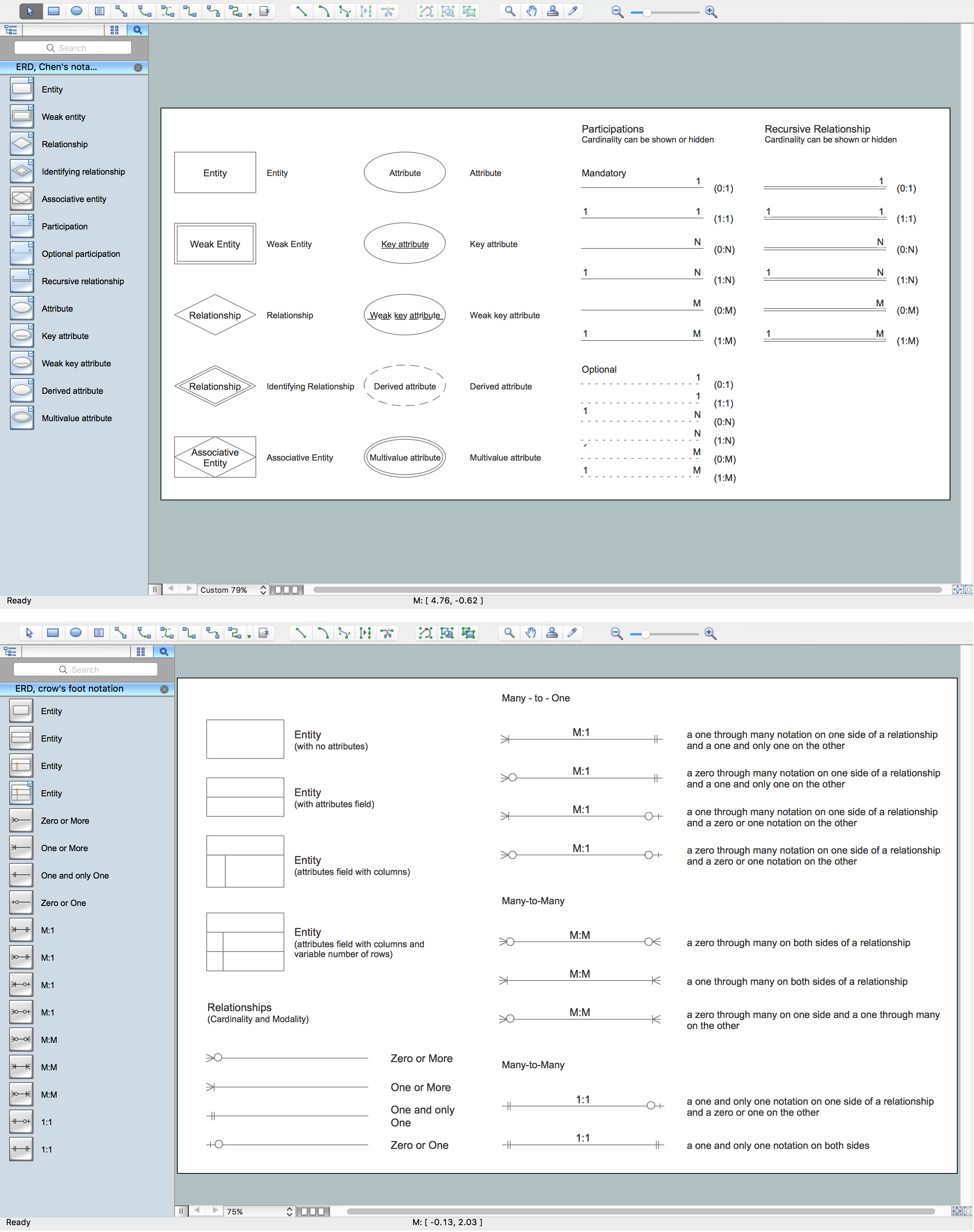Picture: Components of ER Diagram
Related Solution:

### Basic Diagramming →

Perfect charts and graphics diagramming software with rich examples and template. ConceptDraw is ideal to draw Charts and Graphics.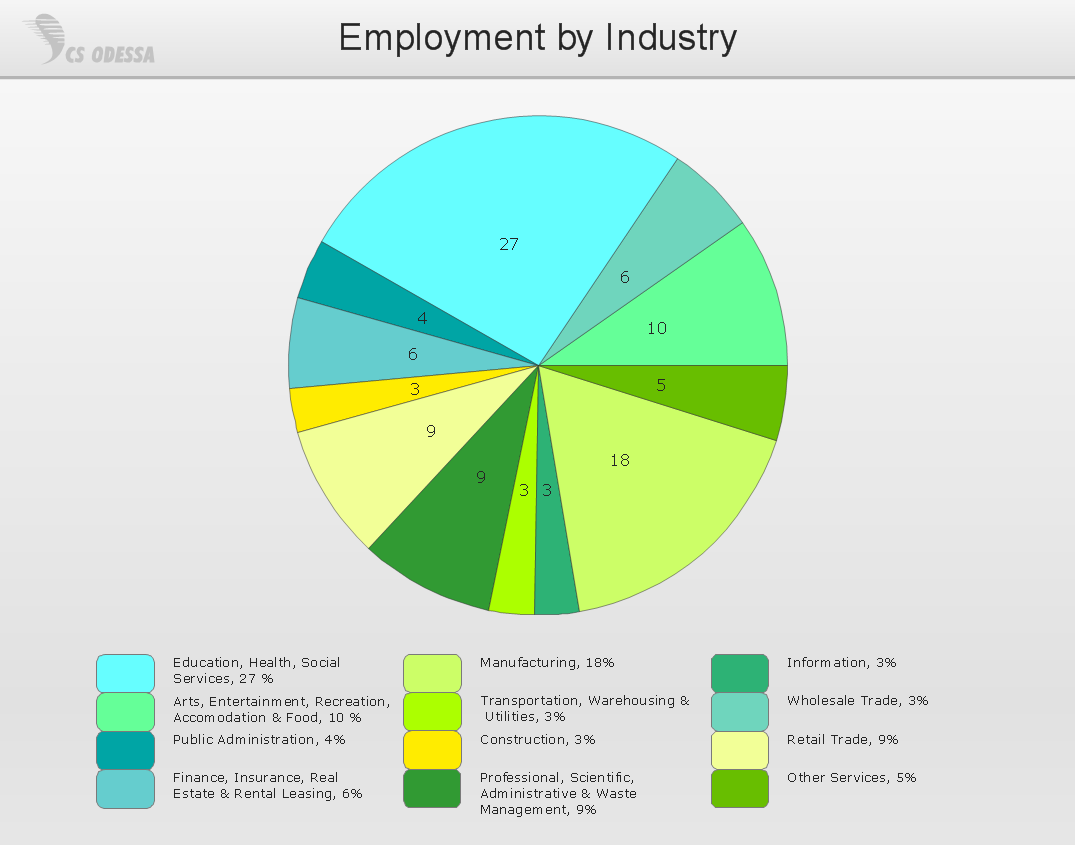Picture: Basic Diagramming
Related Solution:

### Lean Six Sigma Diagram Software →

Lean Six Sigma (LSS) is a well-known in all over the world methodology of quality improvement and business perfection. ConceptDraw DIAGRAM diagramming and vector drawing software is a powerful Lean Six Sigma Diagram Software thanks to the included Value Stream Mapping solution from the Quality Management Area of ConceptDraw Solution Park.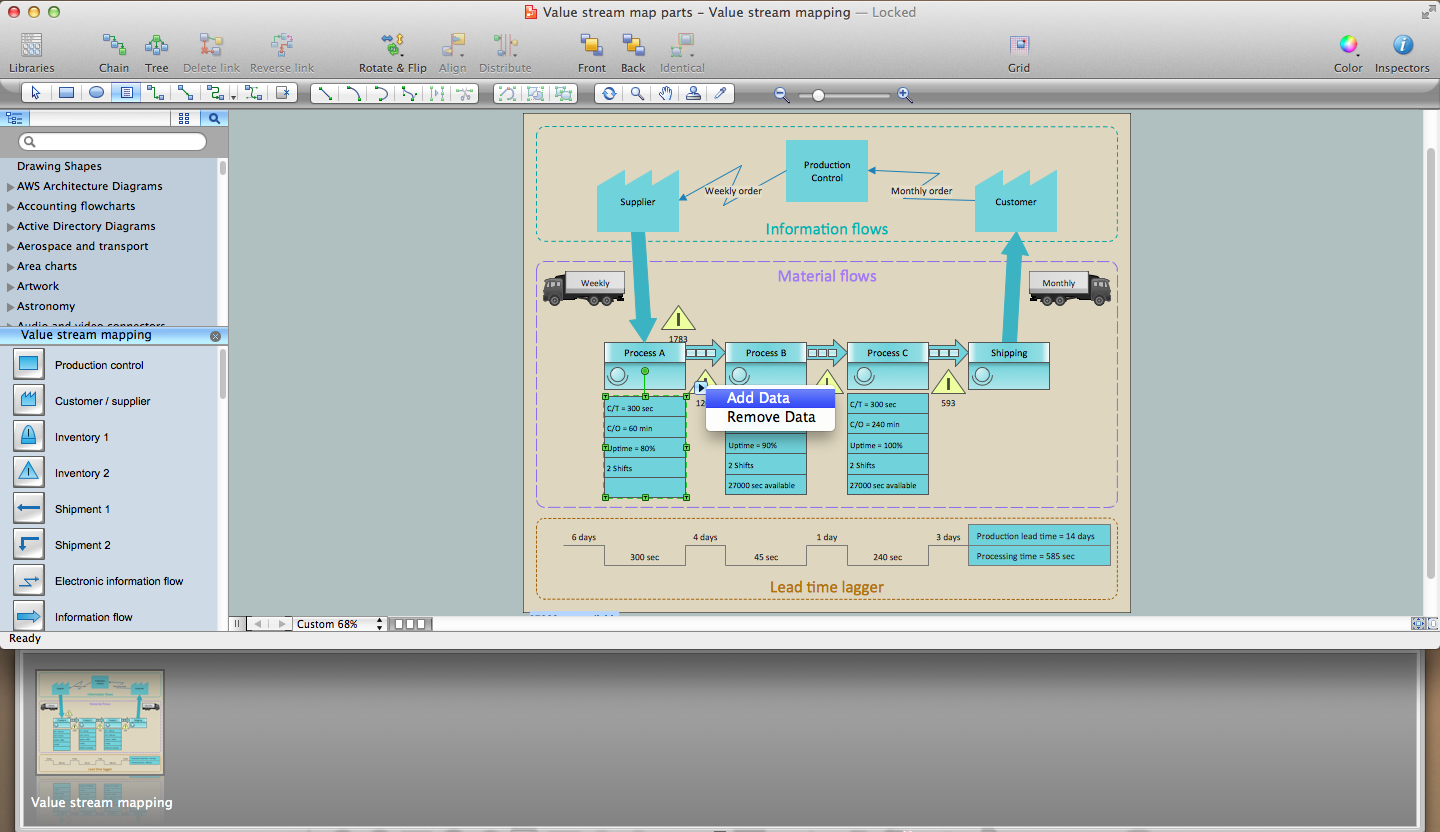Picture: Lean Six Sigma Diagram Software
Related Solution:

### Account Flowchart. Flowchart Examples →

Create your own flow charts of computer or network system account processing using the ConceptDraw DIAGRAM diagramming and vector drawing software extended with the Cross-Functional Flowcharts solution from the Business Processes area of ConceptDraw Solution Park. The flow chart example shows the computer network system account processing.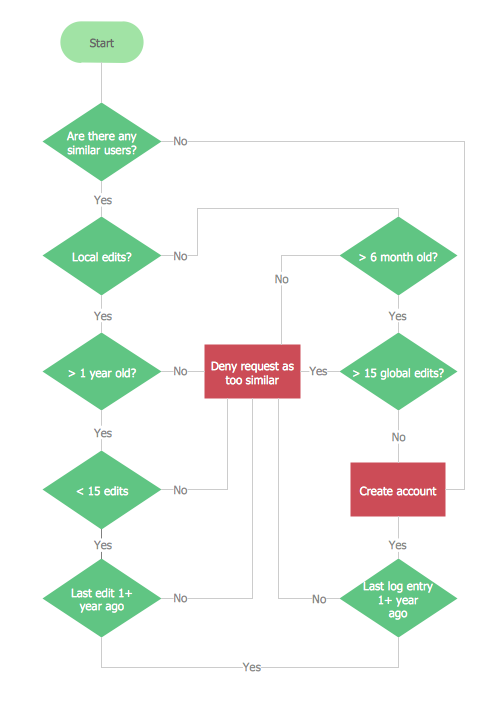Picture: Account Flowchart. Flowchart Examples
Related Solution:

### Block Diagram Software →

The popularity of Block Diagrams grows day by day. The existence of powerful software for their design is an excellent news! ConceptDraw DIAGRAM Block Diagram Software offers the Block Diagrams Solution from the "Diagrams" Area.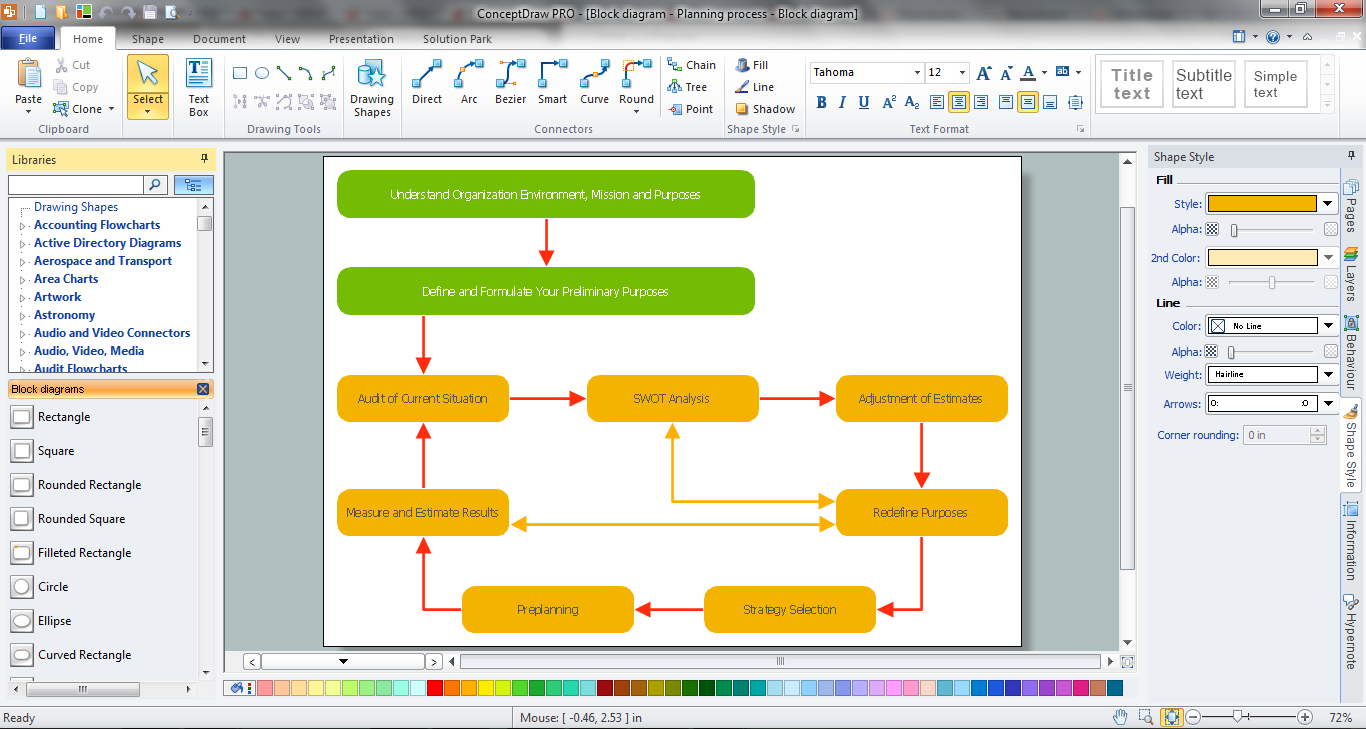Picture: Block Diagram Software
Related Solution:

### Diagram Flow Chart →

ConceptDraw DIAGRAM is a software for producing flow charts. The software delivers built-in object libraries with vector stencils that allows you to use RapidDraw technology. By clicking on direction arrows one can add a new object to flowchart. Users can start drawing their own flowchart diagrams in fast and simple way.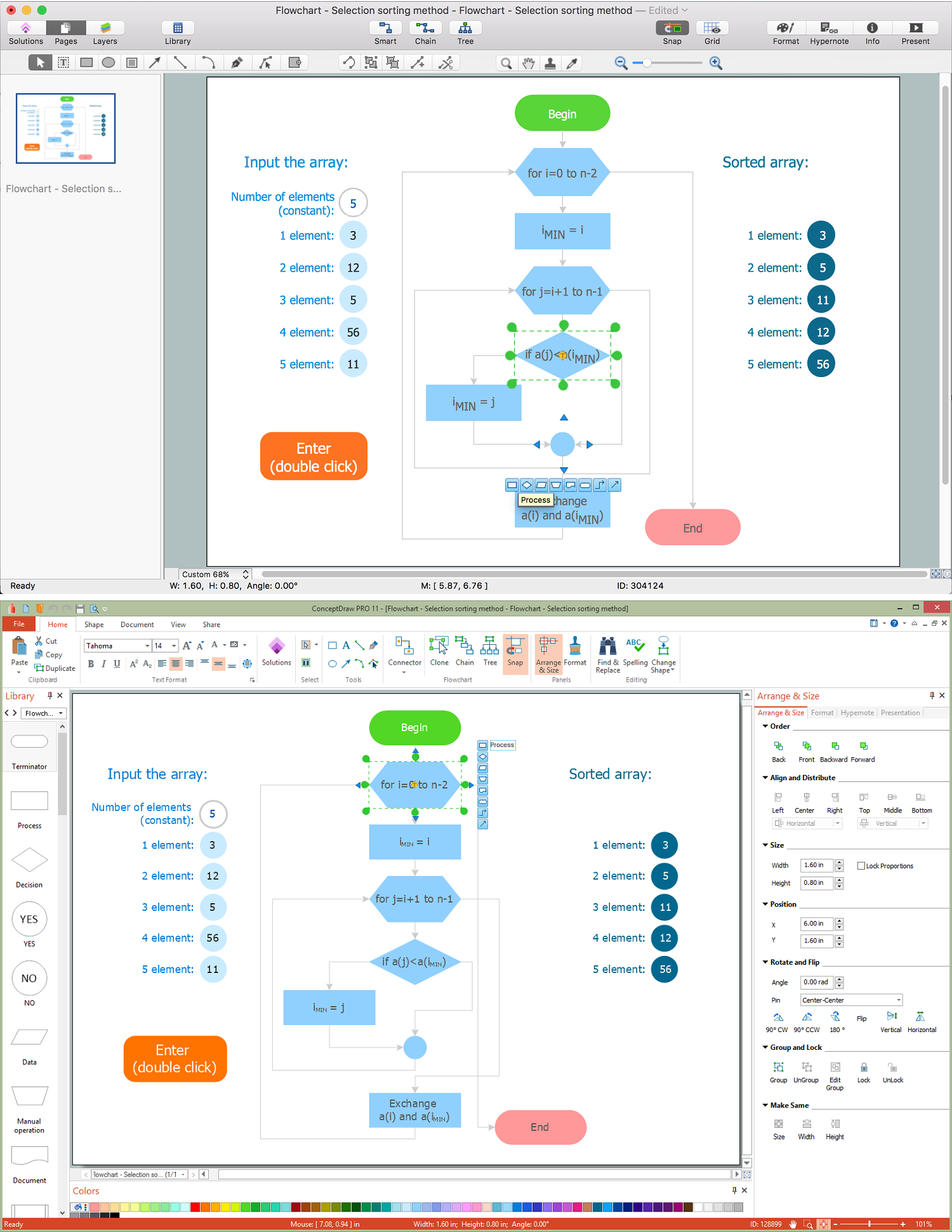Picture: Diagram Flow Chart
Related Solution:

### Venn Diagram Examples for Problem Solving. Computer Science. Chomsky Hierarchy →

A Venn diagram, sometimes referred to as a set diagram, is a diagramming style used to show all the possible logical relations between a finite amount of sets. In mathematical terms, a set is a collection of distinct objects gathered together into a group, which can then itself be termed as a single object. Venn diagrams represent these objects on a page as circles or ellipses, and their placement in relation to each other describes the relationships between them. The Venn diagram example below visualizes the the class of language inclusions described by the Chomsky hierarchy.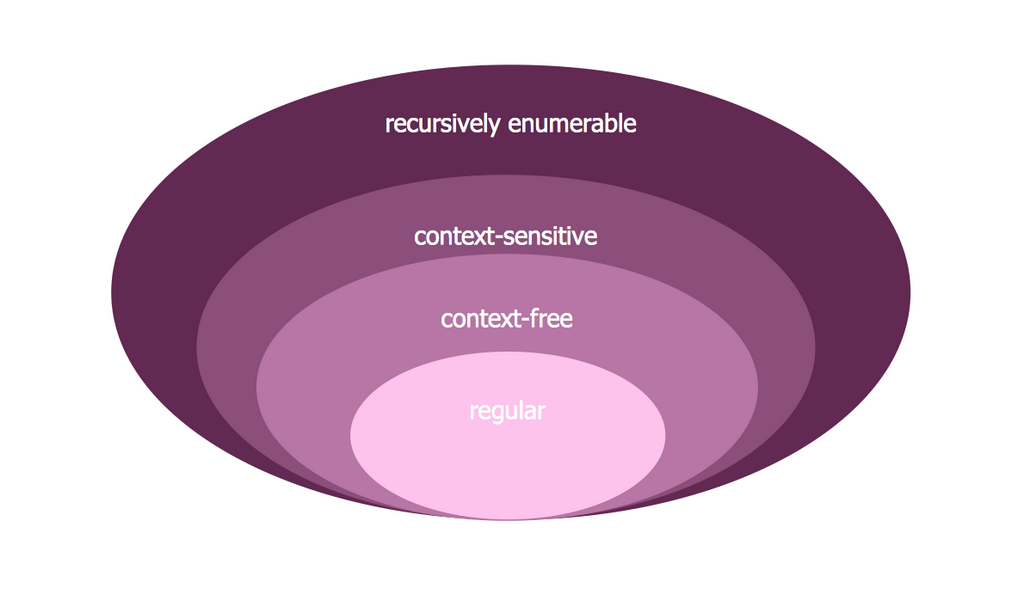Picture: Venn Diagram Examples for Problem Solving. Computer Science. Chomsky Hierarchy
Related Solution:
ConceptDraw
DIAGRAM 14